﻿ 关于我国物价水平的时间序列分析 Time Series Analysis of China’s Price Level

Statistics and Application
Vol.07 No.01(2018), Article ID:23953,7 pages
10.12677/SA.2018.71010

Time Series Analysis of China’s Price Level

Xu Huang

Economic Statistics, Yunnan University of Finance and Economics, Kunming Yunnan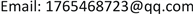Received: Feb. 9th, 2018; accepted: Feb. 21st, 2018; published: Feb. 28th, 2018ABSTRACT

This paper analyzes the CPI index of China from 1985 to 2014 by using time series measurement model. First, the data is analyzed in a stationary manner. It is found that the sequence has an increasing trend through the sequence diagram, so the sequence is a non-stationary sequence  . The sequence is processed by logarithmic process and the first order difference is made. The basic stability is found by the sequence diagram after the difference. Then the ARIMA(p,d,q) model is fitted to the stationary sequence. The fitting effect test and the heteroscedasticity test of the model are carried out   . Finally, the fitting model is used to predict the CPI data of the next 5 years. It can be seen from the prediction results that the CPI is still increasing.

Keywords:CPI Index, ARIMA(p,d,q) Model, Forecast1. 研究背景、意义及目的

2. 模型介绍

$\left\{\begin{array}{l}\varphi \left(B\right){\Delta }^{d}{x}_{t}=\theta \left(B\right){ϵ}_{t}\\ E\left({ϵ}_{t}\right)=0,\text{\hspace{0.17em}}Var\left({ϵ}_{t}\right)={\sigma }_{ϵ}^{2},\text{\hspace{0.17em}}E\left({\epsilon }_{t}{ϵ}_{s}\right)=0,\text{\hspace{0.17em}}s\ne t\\ E\left({\epsilon }_{S}{ϵ}_{t}\right)=0,\text{\hspace{0.17em}}\forall s

${\Delta }^{d}{x}_{t}=\sum _{i=0}^{d}{\left(-1\right)}^{i}{C}_{d}^{i}{x}_{t-i}$

$\Delta {x}_{t}={\gamma }_{1}{x}_{t-1}+\dots +{\gamma }_{p}{x}_{t-p}+{ϵ}_{t},\text{\hspace{0.17em}}\text{\hspace{0.17em}}t=1,2,\cdots ,T$ (1)

$\Delta {x}_{t}=\mu +{\gamma }_{1}{x}_{t-1}+\cdots +{\gamma }_{p}{x}_{t-p}+{ϵ}_{t},\text{\hspace{0.17em}}\text{\hspace{0.17em}}t=1,2,\cdots ,T$ (2)

$\Delta {x}_{t}=\mu +\beta t+{\gamma }_{1}{x}_{t-1}+\cdots +{\gamma }_{p}{x}_{t-p}+{ϵ}_{t},\text{\hspace{0.17em}}\text{\hspace{0.17em}}t=1,2,\cdots ,T$ (3)

${\text{H}}_{0}:{\gamma }_{1}=0$

${\text{H}}_{1}:{\gamma }_{1}\ne 0$

3. 数据来源

4. 实证分析

4.1. 数据处理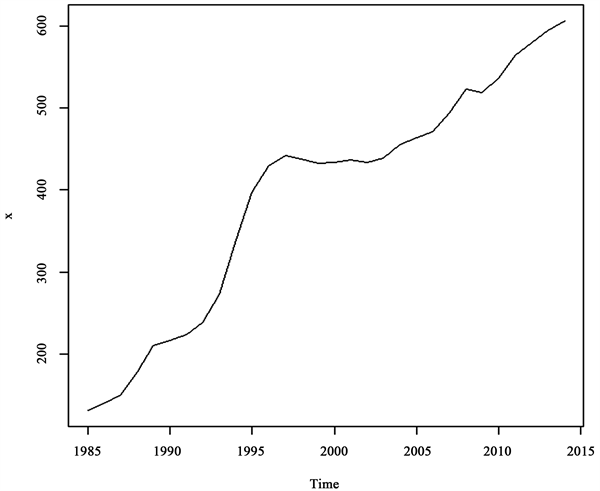Figure 1. 1985-2014 national CPI index (1978 = 100)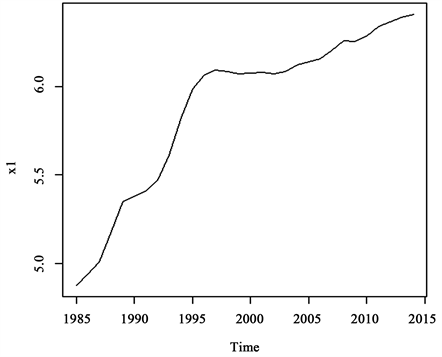Figure 2. 1985-2014 data on the logarithmic data of the national CPI index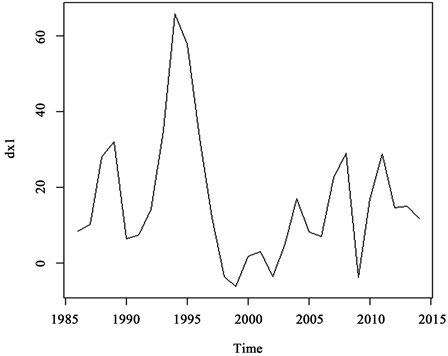Figure 3. First order difference data

4.2. 随机性检验

4.3. 平稳性检验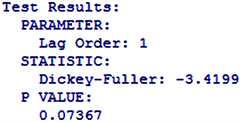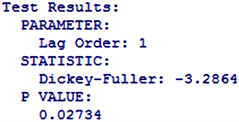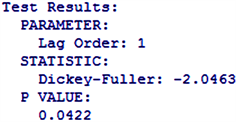Figure 4. ACF inspection figure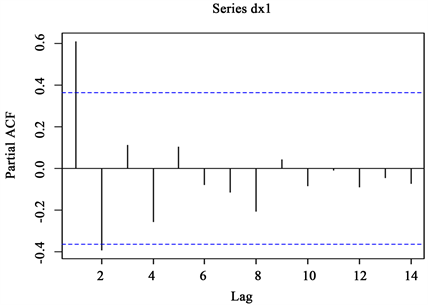Figure 5. PACF inspection figure

4.4. 模型拟合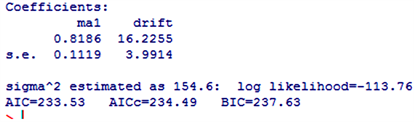${x}_{t}={x}_{t-1}+{\epsilon }_{t}+0.8186{\epsilon }_{t-1}$

4.5. 模型的显著性检验

4.6. 预测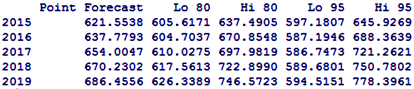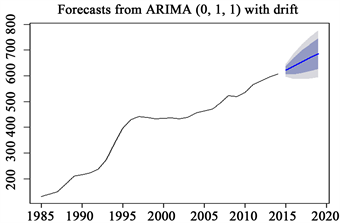Figure 6. CPI forecast for 2015-2020

5. 结论

Time Series Analysis of China’s Price Level[J]. 统计学与应用, 2018, 07(01): 72-78. http://dx.doi.org/10.12677/SA.2018.71010

1. 1. 张鹤. 时间序列分析方法在粮食价格指数分析中的应用[J]. 统计与决策, 2004(9): 32-34.

2. 2. 李可柏, 陈森发. 南通地区月降水量时间序列分析[J]. 数理统计与管理, 2009, 28(3): 555-565.

3. 3. 汤润龙, 颜春莲, 张曙光. 红塔香烟月均价格的时间序列分析[J]. 数理统计与管理, 2005, 24(1): 10-14.

4. 4. 刘颖, 张智慧. 中国人均GDP (1952-2002)时间序列分析[J]. 统计与决策, 2005(2X): 61-62.

5. 5. 姜弘. 居民消费价格指数的时间序列分析及预测[J]. 统计与决策, 2009(4): 117-118.